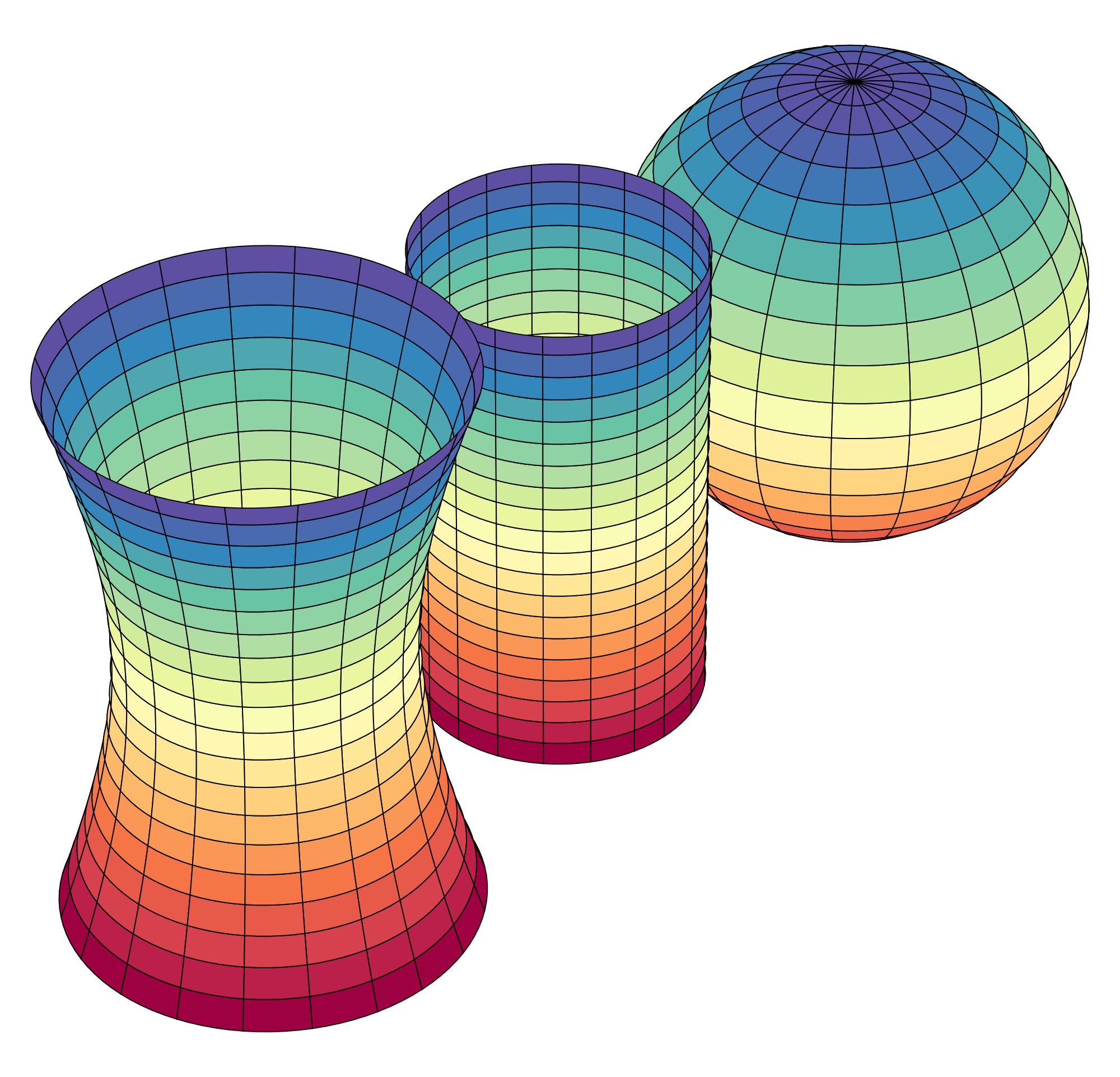# Differential Equations - The Modern Approach

Differential Equations is a sophomore-level course in the calculus sequence, taken after the Calculus II course (integration theory with Taylor Series, Sequences, et al.)

####Upper Division Math Courses

Classical Differential Equations is all about being presented with a type of differential equation, and then learning the paper and pencil techniques to solve the given type differential equation. Classically, you run out of easy differential equations very quickly, and the second half of the course is usually tasked with generalizing functions to Power Series representations, and finding - by hand! - the power series definitions that solve the next level of more difficult differential equation types.

A modern approach to differential equations is forget all of that paper and pencil classical technique - after all, is it really important to learn how to calculate the square root of 3 by hand? Our Modern approach to Differential Equations via the Calculus&Mathematica curriculum is to look at differential equations as what they mean - not how to solve the (rather trivial) cases you can do by hand. Having a powerful tool like Mathematica means you can obtain the solution of a differential equation (if there is one!), but what does the solution mean? How do you interpret it? What does its graph mean? What if the differential equation changes just slightly? What is a driving term?

This modern approach to Differential Equations is a superior approach for physics, economics, and data science. Instead of having a bag of "here's how you solve this differential equation by hand", students leave the course with an appreciation to engage against any differential equation!

Here is a video on the Differential Equation course:

## Distance Calculus - Student ReviewsDate Posted: Apr 13, 2020
Review by: Jorgen M.
Courses Completed: Calculus I
Review: I really enjoyed this course, much more than I thought I would. I needed to finish this course very fast before starting my graduate degree program @ Kellogg. I was able to finish in 3 weeks. I liked the video lectures and the homework process. I highly recommend this course.
Transferred Credits to: Kellogg School of Business, Northwestern UnivDate Posted: Jul 12, 2021
Review by: Henry R.
Courses Completed: Multivariable Calculus
Review: Fantastic and unique course, particularly good for visual/spatial thinkers. The course forces you to develop a real conceptual understanding of the math concepts, as opposed to just teaching math formulas like other courses tend to do. There is a steep learning curve early on to get used to the software and the kind of course this is. Ultimately, I feel like what I learned will allow me to actually use the math and build upon it in the future. If you care about learning, rather than just passing a requirement, this is a great course for you.
Transferred Credits to: Harvard UniversityDate Posted: Jun 21, 2020
Review by: Abdul J.
Courses Completed: Applied Calculus
Review: This was the best class! So much more interesting doing the computer math than a boring lecture class. Diane was so responsive and helpful. I recommend this course.
Transferred Credits to: Villanova University